# LLC 的工作原理（第 I 部分）：电源开关和谐振腔## 概述

LLC 谐振变换器因能满足现代电源设计苛刻的性能要求而成为电力电子领域的热门话题。 LLC 属于庞大的谐振变换器拓扑家族系列，而谐振腔是该拓扑系列的基础特征。谐振腔是一组以特定频率（称为谐振频率）振荡的电感器和电容器组成的电路。

LLC 变换器由 4 个模块组成：电源开关、谐振腔、变压器和二极管整流器（参见图 1）。MOSFET 功率开关首先将输入的直流电压转换为高频方波；随后方波进入谐振腔，由谐振腔消除方波的谐波并输出基频的正弦波；正弦波再通过高频变压器传输到变换器的副边，并根据应用需求对电压进行升压或降压；最后，二极管整流器将正弦波转换为稳定的直流输出。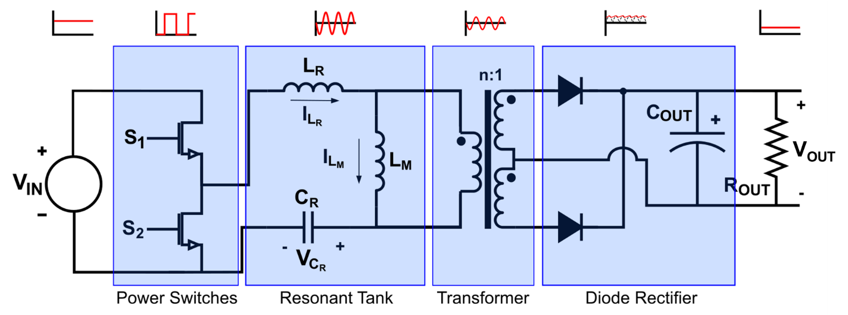LLC 变换器因其谐振特性，即使在非常高的功率下也能保持高效率。该特性同时实现了原边和副边的软开关功能，它降低了开关损耗，从而提高了效率。

## 电源开关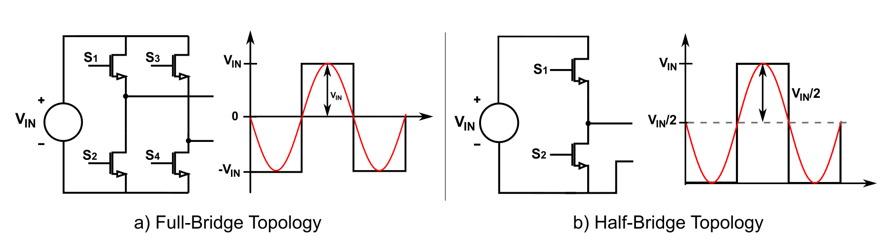## 谐振腔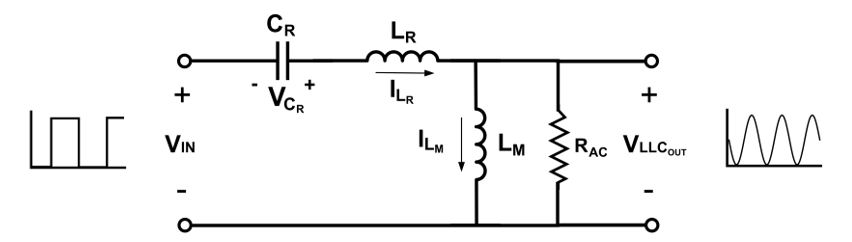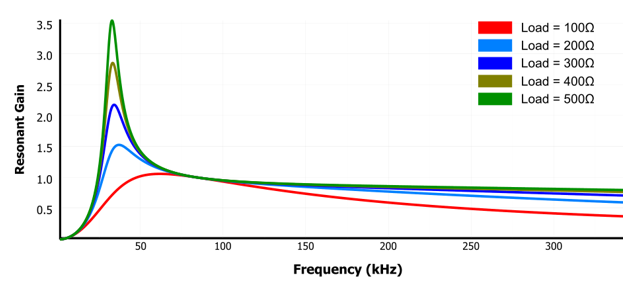LLC 变换器因谐振腔的双电感器而具有宽工作范围与高效率。要了解其原理，首先要了解谐振腔采用不同电感器时，在重载和轻载条件下的响应。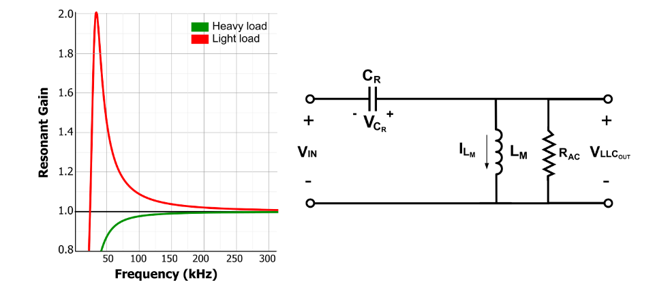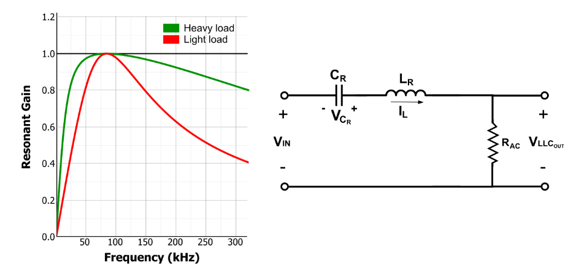$$f_{R} = \frac {1}{2π \sqrt {L_{R} \times C_{R}}}$$ $$f_{M} = \frac {1}{2π \sqrt {L_{M}+L_{R} \times C_{R}}}$$

$$R_{AC} = \frac {8xn^2}{π} \times R_{O}$$ $$Q = \frac {\sqrt {L_{R}/C_{R}}}{R_{AC}}$$

$$f_{N} = \frac {f_{SW}}{f_{R}}$$

$$L_{N} = \frac {L_{M}}{L_{R}}$$

$$M_{G}(Q,Ln,Fn) = \frac {V_{OUT[AC]}}{V_{IN[AC]}} = \frac {f_{N}^2 \times (L_{N}-1)}{(f_{N}^2-1)^2 + f_{N}^2 x(f_{N}^2 - 1) \times (L_{N}-1)^2 \times Q^2}$$

## 软开关

LLC 变换器的另一个常见特性是其软开关能力。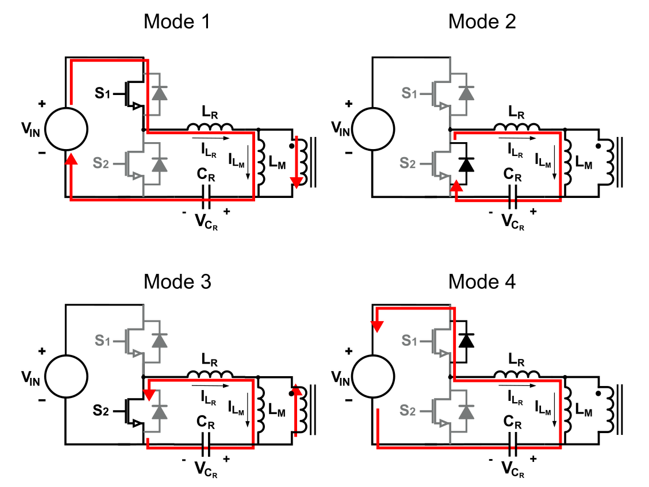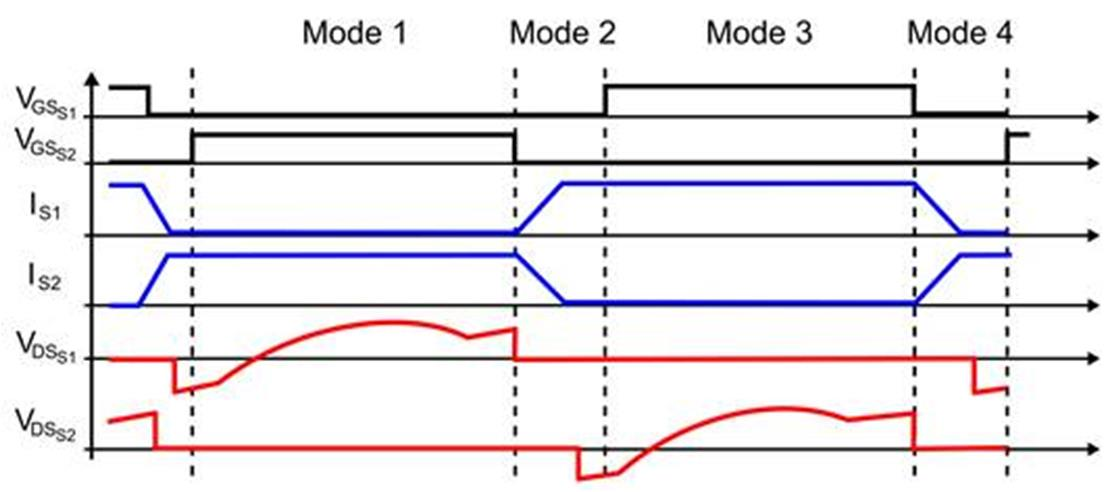## 结论

MPS 的 LLC Designer 等设计工具是加速 LLC 设计过程的理想选择，它允许您快速迭代不同的增益和频率，并计算出设计所需的组件值。